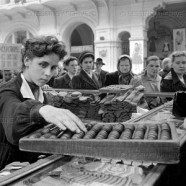# CalculatorsThis section includes animated displays of computation using the Iconic Calculator. Each of the three modes shows a different number system.  All use depth-value notation implemented as 2D container numbers. The Image is of the GOM department store, the largest store in Moscow, in 1956. The clerk is calculating a customer’s bill using a large abacus, apparently the state of the art in cash registers at the time.

## Section Contents

The Iconic Calculator shows dynamic animations of iconic computation in three modes: binary, decimal using discrete units, and decimal using numerals.

The Binary Calculator shows the addition and subtraction of binary numbers .

The Decimal Unit Calculator shows the addition and subtraction of decimal unit numbers.

The Decimal Digit Calculator shows the addition and subtraction of decimal digit numbers. This calculator closely resembles our current arithmetic system, except that it uses spatial/iconic rather than linear/textual principles of computation.

The first animation on each page shows the entire sequence of entering a problem and computing the solution.  The rest of the animations show only the Iconic Arithmetic Calculator display screen while suppressing the keyboard and video controls.